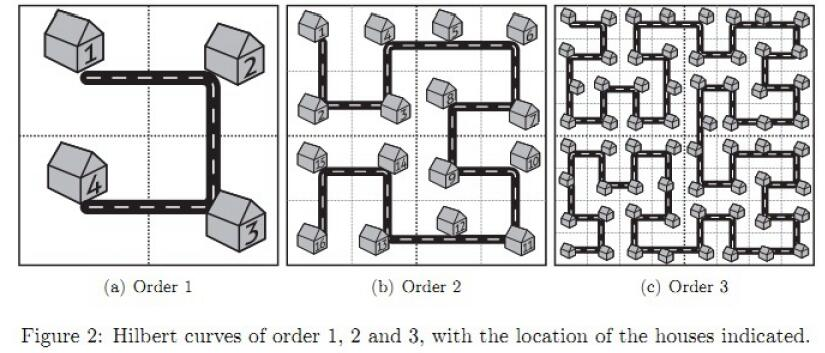upc 奇怪的道路 分形之城---改版

[提交] [状态]2 4
2 1 3 1
3 2 2 2
2 3 3 3
3 4 2 4

13
11
1
3

(1)从第2阶开始，每一阶都是由前面一阶的图形通过不同的旋转构成的。

(2)若每一阶可以分为 4 个大小相同的方块 ，那么每个方块中点的数量为 1 << (n * 2 - 2) 个，边长为 1 << (n - 1) 。

(1) 当坐标落在第一个方块，递归处理 cal(y ,x ,n-1)。
(2) 当坐标落在第二个方块，递归处理 bloc + cal(x ,y-l ,n-1)。
(3) 当坐标落在第三个方块，递归处理 bloc × 2 + cal(x-l ,y-l ,n-1)。
(4) 当坐标落在第四个方块，递归处理 bloc × 3 + cal(l-y-1 ,l-(x-l)-1 ,h-1)

```#include

#include

#include

#include

using namespace std;typedef long long LL;typedef pair

PII;int t,n;int cal(int x,int y,int h){

if(h==1)
{

if(x==0&&y==0) return 1;
if(x==0&&y==1) return 2;
if(x==1&&y==1) return 3;
if(x==1&&y==0) return 4;
}
int bloc=1ll<<(2*h-2),l=1ll<<(h-1);
if(x

if(x

=l) return bloc+cal(x,y-l,h-1);
if(x>=l&&y>=l) return bloc*2+cal(x-l,y-l,h-1);
if(x>=l&&y

scanf("%d%d",&n,&t);
while(t--)
{

int x1,y1,x2,y2;
scanf("%d%d%d%d",&x1,&y1,&x2,&y2);
x1--,x2--,y1--,y2--;
int a=cal(x1,y1,n);
int b=cal(x2,y2,n);//
cout<
<<' '<<

printf("%d\n",abs(a-b));
}
return 0;}

```

#### 最新留言

[***.238.104.143]2022年07月07日 15时26分34秒

## 关于作者喝酒易醉，品茶养心，人生如梦，品茶悟道，何以解忧？唯有杜康！
-- 愿君每日到此一游！